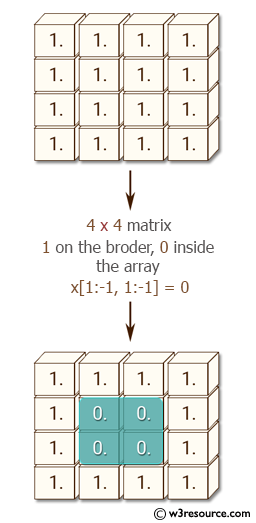﻿ NumPy: Create a 10x10 matrix, in which the elements on the borders will be equal to 1, and inside 0 - w3resource# NumPy: Create a 10x10 matrix, in which the elements on the borders will be equal to 1, and inside 0

## NumPy: Basic Exercise-28 with Solution

Write a NumPy program to create a 10x10 matrix, in which the elements on the borders will be equal to 1, and inside 0.

Sample Solution :

Python Code :

``````import numpy as np
x = np.ones((10, 10))
x[1:-1, 1:-1] = 0
print(x)
``````

Sample Output:

```[[ 1.  1.  1.  1.  1.  1.  1.  1.  1.  1.]
[ 1.  0.  0.  0.  0.  0.  0.  0.  0.  1.]
[ 1.  0.  0.  0.  0.  0.  0.  0.  0.  1.]
[ 1.  0.  0.  0.  0.  0.  0.  0.  0.  1.]
[ 1.  0.  0.  0.  0.  0.  0.  0.  0.  1.]
[ 1.  0.  0.  0.  0.  0.  0.  0.  0.  1.]
[ 1.  0.  0.  0.  0.  0.  0.  0.  0.  1.]
[ 1.  0.  0.  0.  0.  0.  0.  0.  0.  1.]
[ 1.  0.  0.  0.  0.  0.  0.  0.  0.  1.]
[ 1.  1.  1.  1.  1.  1.  1.  1.  1.  1.]]
```

Pictorial Presentation:Python Code Editor:

Have another way to solve this solution? Contribute your code (and comments) through Disqus.

What is the difficulty level of this exercise?

Test your Python skills with w3resource's quiz

﻿

## Python: Tips of the Day

Merges two or more lists into a list of lists, combining elements from each of the input lists based on their positions

Example:

```def tips_merge(*args, fill_value=None):
max_length = max([len(lst) for lst in args])
result = []
for i in range(max_length):
result.append([
args[k][i] if i < len(args[k])
else fill_value for k in range(len(args))
])
return result
print(tips_merge(['x', 'y'], [1, 2], [True, False]))
print(tips_merge(['x'], [1, 2], [True, False]))
print(tips_merge(['x'], [1, 2], [True, False], fill_value = '_'))
```

Output:

```[['x', 1, True], ['y', 2, False]]
[['x', 1, True], [None, 2, False]]
[['x', 1, True], ['_', 2, False]]
```